1. Scale of the map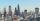The distance between two cities is actually 30 km and the map is 6 cm. What is the scale of the map?
2. Guppies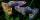Audrey has some guppies in a fish tank. The ration of the oranges guppies to silver guppies is 3:5. She has 12y oranges guppies. Write the number of silver guppies she has in terms of y
3. Two bricks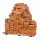One brick weighs 1kg and half a brick, how many weigh two bricks?
4. Crates 2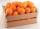One crate will hold 50 oranges. If Bob needs to ship 932 oranges, how many crates will he need?
5. PexesoDrawing paper has dimensions of 60cm and 840mm. Pupils have to divide it into squares so that they can make a pexeso. What dimension must squares have if their side is to be larger than 3cm and less than 10cm?
6. Prime factorsWrite 98 as product of prime factors
7. Volume of cone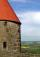Find the volume of a right circular cone-shaped building with a height of 9 cm and a radius base of 7 cm.
8. Curved surface area CSA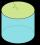A cylinder 5cm high has a base radius(7/2) cm. Calculate the curved surface area.
9. Mixed2improperWrite the mixed number as an improper fraction. 166 2/3
10. Sum of fractionsWhat is the sum of 2/3+3/5?
11. Sewing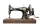The lady cut off one half of cloth. She needed three-quarters of this piece to sew a skirt. What part of the original piece of cloth still remained?
12. If one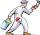If one litre of pant covers an area of 5 m2 how much paint is needed to cover: a) rectangular swimming pool With dimensions 4m x 3m x 2.5m (the Inside walls and the floor only) b) the Inside walls and floor of a cylindrical reservoir with diameter 3m andThe railroad runs parallel to the railway. The train travels at 36 km/h on track. In the opposite direction, the motorcycle rides at 90 km/h. The train passes in 3 seconds. How long is a train?
14. RectanglesHow many different rectangles with sides integers (in mm) have a circumference exactly 1000 cm?
15. The cubeThe cube has an edge of 25 cm. We cut it into small cubes of 5 cm long side. How many of these little ones left when we build a new cube of 20 cm in length?
16. Simple equation 5Solve equation with fractions: X × 3/8 = 1/2
17. Square into three rectangles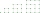Divide the square with a side length of 12 cm into three rectangles with have the same circumference so that these circumferences are as small as possible.
18. Clothes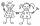Danka and Janka collect clothes. Danka had 9 more than Janka, so she gave her 7. Which now has more cloth and how many?
19. SymbolsIf 2*3 = 60 ; 3*4 = 120 and 4*5 = 200, what is 2*5?
20. One hectareHow many square meters are one hectare?

Do you have an interesting mathematical word problem that you can't solve it? Enter it, and we can try to solve it.

To this e-mail address, we will reply solution; solved examples are also published here. Please enter the e-mail correctly and check whether you don't have a full mailbox.

Please do not submit problems from current active competitions such as Mathematical Olympiad, correspondence seminars etc...